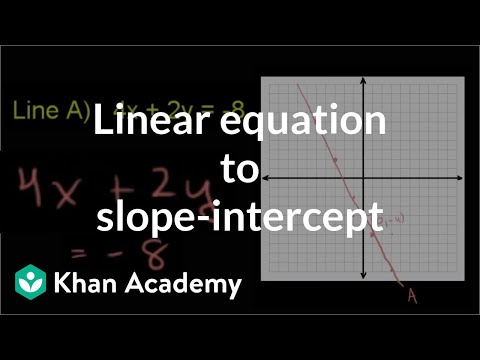Video

# Testing solutions to linear equations (Full video)

Khan Academy

## Supporting grades: 8

Description: Sal matches a table of values and an equation that both model the same relationship. Which equation was used to generate this table? And these points have been generated by one of these equations right over here, so let's just see which of these equations actually could have generated all of these points. So let's go to this first point right over here, 4 comma negative 8, and let's go equation by equation.

You must log inorsign upif you want to:*

*Teacher Advisor is 100% free.

### Other videos you might be interested in### Ratio and Proportion in Grades 6-8: Connections to College and Career Skills (Full video)

#### The Hunt Institute